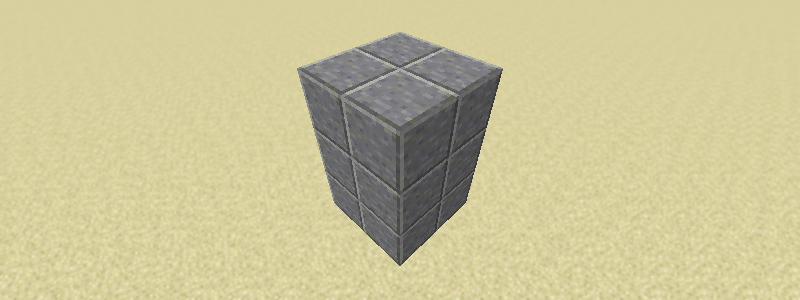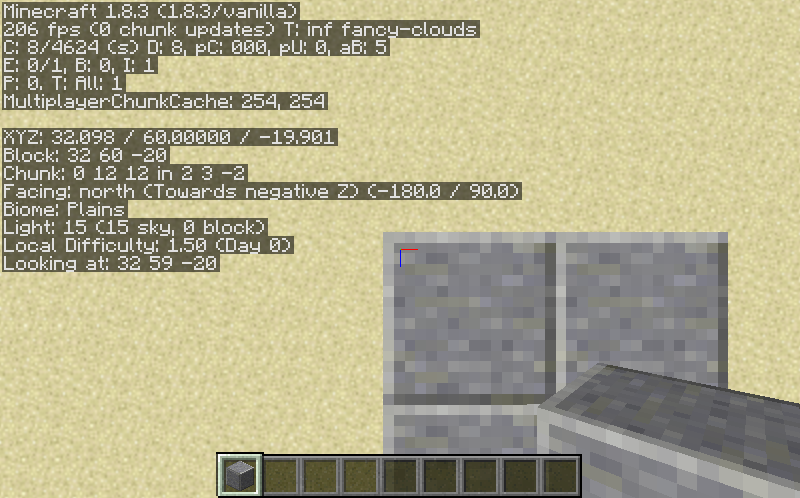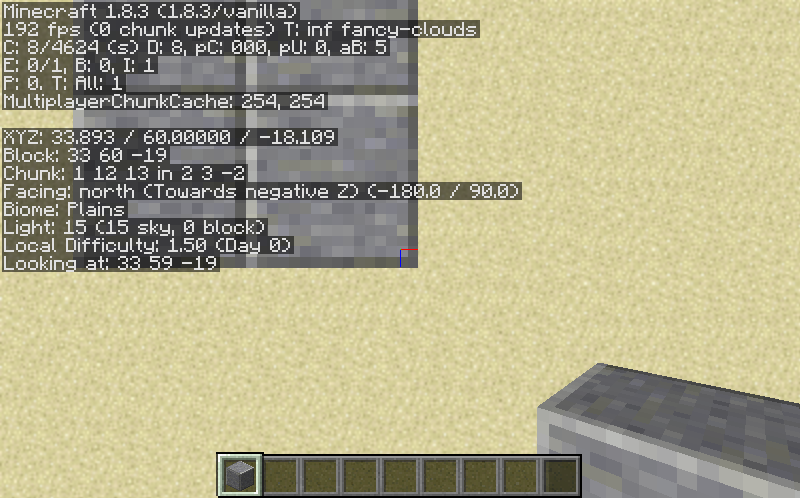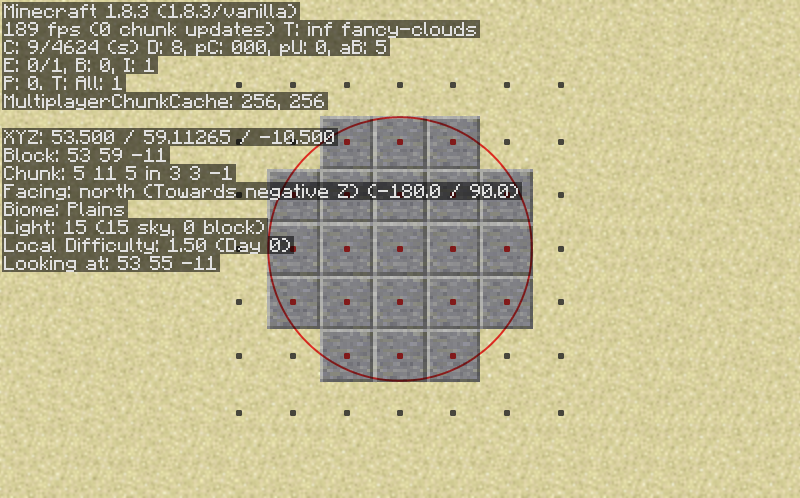# Guides XML Pointers

First of all, region coordinates in PGM are real numbers. That means they can be fractional values, like 2.3, 4.5, 6.789, and so on. A coordinate represents a point on one of the three axes (X, Y, or Z), and a set of three coordinates represents a point in 3D space. Coordinates do not represent blocks, at least not directly. When PGM needs to decide if a block is inside a region, it checks if the point at the center of the block is inside the region. The center point of a block will always have coordinates that end in `.5`. When making regions, you have to make sure that all the block centers are inside the region.

Here is an example. Let’s say we want to make region for a destroyable that looks like this:First, we stand very close to one corner of the region and note the coordinates are `(32, 60, -20)`:Then we stand over the opposite corner and note the coordinates are `(34, 60, -18)`.
We know the monument is three blocks tall, so we’ll just subtract three from the Y coordinate to get `(34, 57, -18)`:Notice that we are rounding to the nearest integer, since those will obviously be the coordinates of the corner we are standing close to. We don’t need to worry about positive vs negative coordinates, as they work the same way, and we never need to add or subtract one.

With these coordinates, we can make a cuboid region for the monument:

``````<cuboid min="32, 60, -20" max="34, 57, -18"/>
``````

For style points, we can make sure all the low coordinates are in min and the high ones in max:

``````<cuboid min="32, 57, -20" max="34, 60, -18"/>
``````

This is not necessary, as PGM will fix it for you, but it might make the XML easier for a human to read and edit.

An easy way to check that cuboid regions are correct is to simply subtract the low coordinates from the high ones. The result should be the size of the region. If it isn’t, you did something wrong.

Let’s try a more interesting region: a cylinder. A cylinder is defined by its base (center) point, radius, and height. Assuming one layer of blocks, the cylinder in the image below is based at `(53.5, 57, -10.5)` and has a radius of `2.5` and a height of `1`. Blocks with their center point inside the cylinder will be considered part of the region. I’ve highlighted the region boundary and the block centers so you can easily see how this works:So the region would be:

``````<cylinder base="53.5, 57, -10.5" radius="2.5" height="1"/>
``````

### Regions: The Wrong Way

I know that many of you are using WorldEdit to get region coordinates, and my advice to you is DON’T . WorldEdit uses a dumb coordinate system that will not give you correct regions, and while it’s possible to convert the coordinates, it’s going to confuse you and cause mistakes, and it’s just not worth the trouble. I suspect that WorldEdit is the reason we have so many region errors in our map collection right now.